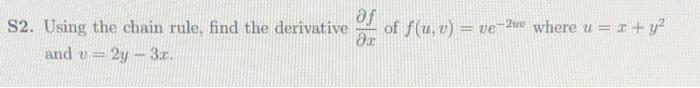### Create an Account

Already have account?

### Forgot Your Password ?

Home / Questions / af S2. Using the chain rule, find the derivative of f(u, v) = ve-24 where u = x + y2 Эх an...

# af S2. Using the chain rule, find the derivative of f(u, v) = ve-24 where u = x + y2 Эх and y = 2y - 3

af S2. Using the chain rule, find the derivative of f(u, v) = ve-24 where u = x + y2 Эх and y = 2y - 3Apr 15 2021 View more View Less

#### Answer (Solved)Subscribe To Get Solution